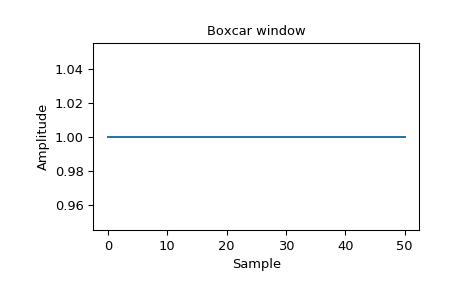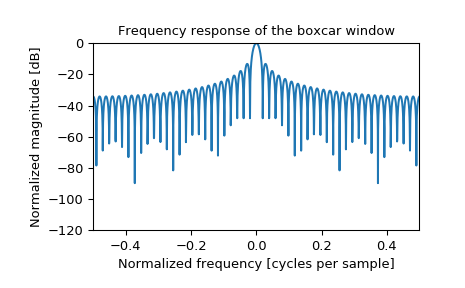# scipy.signal.windows.boxcar¶

scipy.signal.windows.boxcar(M, sym=True)[source]

Return a boxcar or rectangular window.

Also known as a rectangular window or Dirichlet window, this is equivalent to no window at all.

Parameters
Mint

Number of points in the output window. If zero or less, an empty array is returned.

symbool, optional

Whether the window is symmetric. (Has no effect for boxcar.)

Returns
wndarray

The window, with the maximum value normalized to 1.

Examples

Plot the window and its frequency response:

>>> from scipy import signal
>>> from scipy.fftpack import fft, fftshift
>>> import matplotlib.pyplot as plt

>>> window = signal.boxcar(51)
>>> plt.plot(window)
>>> plt.title("Boxcar window")
>>> plt.ylabel("Amplitude")
>>> plt.xlabel("Sample")

>>> plt.figure()
>>> A = fft(window, 2048) / (len(window)/2.0)
>>> freq = np.linspace(-0.5, 0.5, len(A))
>>> response = 20 * np.log10(np.abs(fftshift(A / abs(A).max())))
>>> plt.plot(freq, response)
>>> plt.axis([-0.5, 0.5, -120, 0])
>>> plt.title("Frequency response of the boxcar window")
>>> plt.ylabel("Normalized magnitude [dB]")
>>> plt.xlabel("Normalized frequency [cycles per sample]")